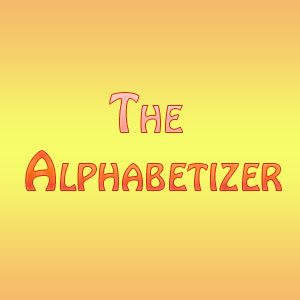• A = ALPHA
• B = BRAVO
• C = CHARLIE
• D = DELTA
• E = ECHO
• F = FOXTROT
• G = GOLF
• H = HOTEL
• I = INDIA
• J = JULIET
• K = KILO
• L = LIMA
• M = MIKE
• N = NOVEMBER
• O = OSCAR
• P = PAPA
• Q = QUEBEC
• R = ROMEO
• S = SIERRA
• T = TANGO
• U = UNIFORM
• V = VICTOR
• W = WHISKEY
• X = X-RAY
• Y = YANKEE
• Z = ZULU
• ALPHA
• BRAVO
• CHARLIE
• DELTA
• ECHO
• FOXTROT
• GOLF
• HOTEL
• INDIA
• JULIET
• KILO
• LIMA
• MIKE
• NOVEMBER
• OSCAR
• PAPA
• QUEBEC
• ROMEO
• SIERRA
• TANGO
• UNIFORM
• VICTOR
• WHISKEY
• X-RAY
• YANKEE
• ZULU

### Related ResourcesThe Alphabetizer online app can be used to sort the words of the phonetic military alphabet which is used by pilots and servicemen to communicate via radio in the military. Whiskey Tango Foxtrot – WTF